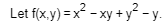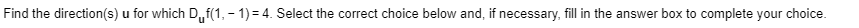# Finding Directional Derivative

Homework Statement:
see post
Relevant Equations:
∇f⋅u= Direction vectorThe gradient is < (2x-y), (-x+2y-1) >
at P(1,-1) the gradient is <3, -4>

Since ∇f⋅u= Direction vector, it seems that we should set the equation equal to the desired directional derivative.

< 3, -4 > ⋅ < a, b > = 4

which becomes

3a-4b=4

I thought of making a list of possible combinations of a's and b's which satisfy this equation like so

a, b
corresponding direction vector

0, 1
<0, 1>

(4/3), 0
(for which there is no direction vector??)

2, (1/2)
< 4/√17 , 1/√17 >

But it seems that there are an infinite number of possible combinations. And the question is asking for all of them.

timetraveller123
i am not sure but i don't think it can be infinite in two dimension
i think it is two
##
(3,-4) , (a,b) = 5.1.cos \theta = 4
##
there is two values of theta one negative of the other. maybe in three dimension you might have infinite vectors because they all in a cone of same theta. but in two dimension i think it might be two vectors

Staff Emeritus
Homework Helper
Gold Member
Problem Statement: see post
Relevant Equations: ∇f⋅u= Direction vector

View attachment 244655View attachment 244656

The gradient is < (2x-y), (-x+2y-1) >
at P(1,-1) the gradient is <3, -4>

Since ∇f⋅u= Direction vector, it seems that we should set the equation equal to the desired directional derivative.

< 3, -4 > ⋅ < a, b > = 4

which becomes

3a-4b=4

I thought of making a list of possible combinations of a's and b's which satisfy this equation like so

a, b
corresponding direction vector

0, 1
<0, 1>

(4/3), 0
(for which there is no direction vector??)

2, (1/2)
< 4/√17 , 1/√17 >

But it seems that there are an infinite number of possible combinations. And the question is asking for all of them.